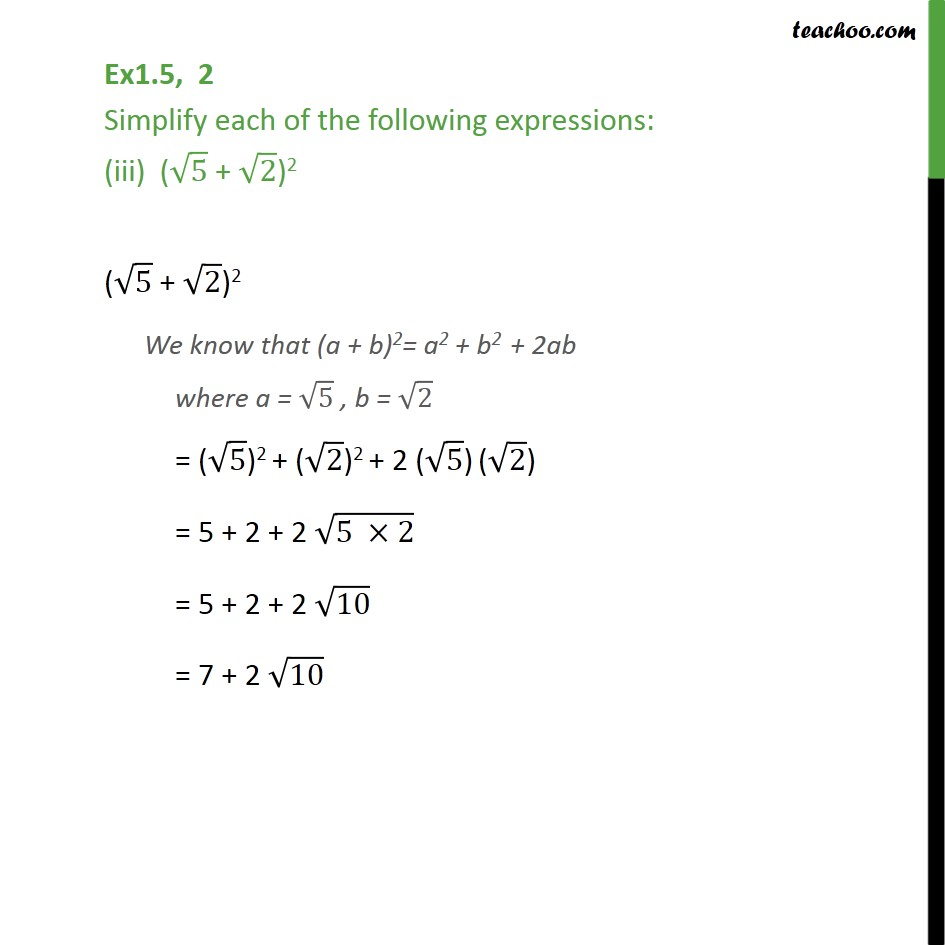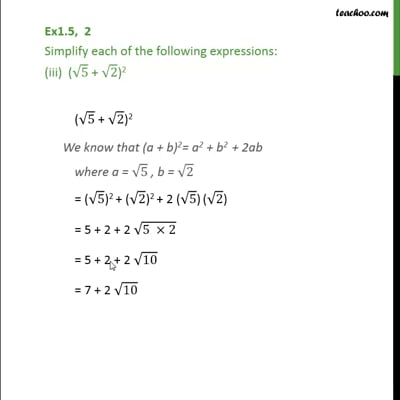Ex 1.5

Chapter 1 Class 9 Number Systems
Serial order wiseThis video is only available for Teachoo black users

Maths Crash Course - Live lectures + all videos + Real time Doubt solving!

### Transcript

Ex1.5, 2 Simplify each of the following expressions: (iii) ( 5 + 2)2 ( 5 + 2)2 We know that (a + b)2= a2 + b2 + 2ab where a = 5 , b = 2 = ( 5)2 + ( 2)2 + 2 ( 5) ( 2) = 5 + 2 + 2 (5 2) = 5 + 2 + 2 10 = 7 + 2 10